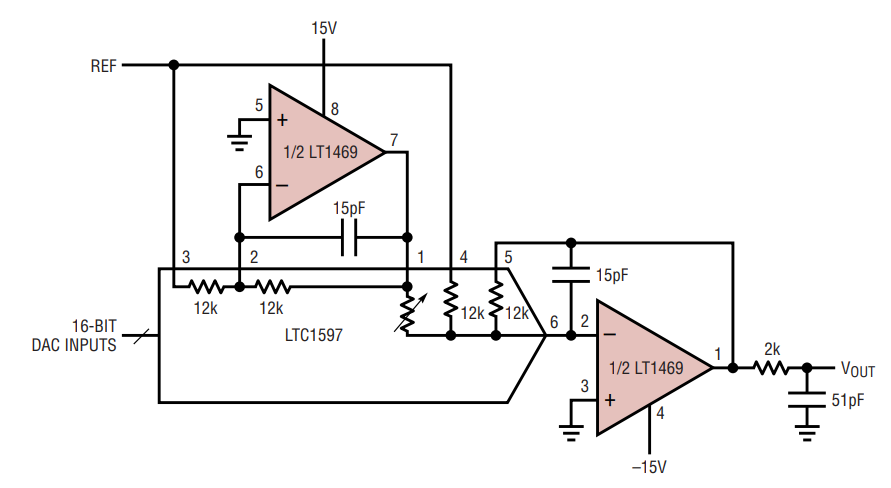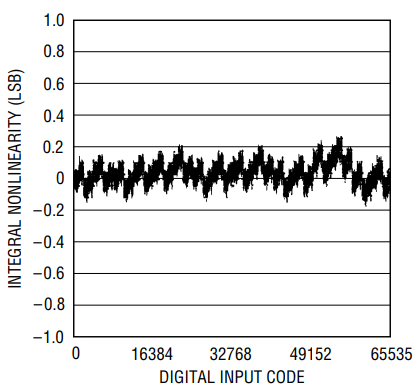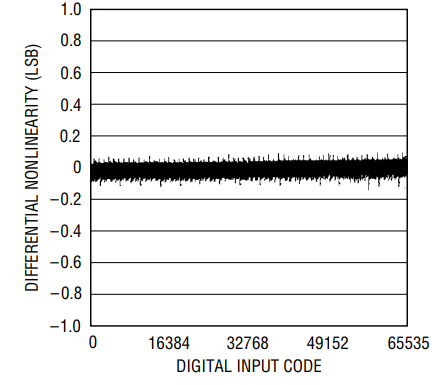Dual Operational Amplifier Combines 16-Bit Precision with High Speed

Introduction

The LT1469 is a dual operational amplifier that has been optimized for accuracy and speed in 16-bit systems. The amplifier settles in just 900ns to 150µV for a 10V step. The LT1469 also features the excellent DC specifications required for 16-bit designs. Input offset voltage is 125µV maximum, input bias current is 10nA maximum for the inverting input and minimum DC gain is 300V/mV. The LT1469 specifications are summarized in Table 1.

 Parameter Value Input Offset Voltage 125µV (Max) Inverting Input Bias Current 10nA (Max) Noninverting Input Bias Current 40nA (Max) DC Gain 300V/mV (Min) CMRR 96dB (Min) PSRR 100dB (Min) Channel Separation 100dB (Min) Input Noise Voltage 5nV/√Hz Input Noise Current 0.6pA /√Hz Gain Bandwidth 90MHz Slew Rate 22V/µs Settling Time (AV = –1, 150µV, 10V Step) 900ns Settling Time (with LTC1597, CF = 15pF, 20V Step) 2.4µs THD for 10VP-P, 100kHz –96.5dB Supply Current, VS = ±15V (Per Amplifier) 5.2mA (Max)

This article presents two applications of the LT1469 in 16-bit data-conversion systems. The first application is with a fast current-output digital-to-analog converter (DAC), such as the LTC1597. The dual LT1469 amplifier allows this DAC to operate in bipolar, 4-quadrant multiplying mode. The second application illustrates the use of this dual amplifier as a buffer for a differential analog-to-digital converter (ADC), such as the 333ksps LTC1604.

16-Bit 4-Quadrant DAC with 2.4µs Settling Time

The fastest, most precise way to achieve 16-bit digital-to-analog conversion is using a current-output DAC followed by a precision amplifier for current-to-voltage conversion. Figure 1 shows the LT1469 used in conjunction with the LTC1597 16-bit current-output DAC. The first amplifier is used as the current-to-voltage (I/V) converter at the output of the DAC. The second amplifier is used to invert the reference input voltage. All the resistors are internal to the DAC and precisely trimmed. With a fixed 10V reference input (such as could be provided by the LT1021-10), the reference inversion allows a bipolar output swing, that is, from –10V to 10V. In addition, because of the high bandwidth and low distortion of the LT1469, this configuration allows the reference input to be a variable signal, such as a sine wave, for full 4-quadrant multiplication operation. Figure 2 shows signal-to-(noise plus distortion) measurement results of this circuit.Figure 1. 16-bit DAC I/V converter and reference inverter.Figure 2. Signal to (noise plus distortion) for Figure 1’s circuit (code = all zeros).

The key AC specification of the circuit in Figure 1 is settling time, since this limits the DAC update rate. In an optimum configuration, the settling time of the LT1469 alone is a blistering 900ns. In Figure 1, settling time is limited by the need to compensate for the DAC output capacitance, which, for the LTC1597, varies from 70pF to 115pF, depending on the input code. This capacitance at the amplifier’s inverting input combines with the internal feedback resistor to form a zero in the closed-loop frequency response in the vicinity of 100kHz–200kHz. Without a feedback capacitor, the circuit will oscillate. A 15pF feedback capacitor stabilizes the circuit by adding a pole at 880kHz. This 12kΩ||15pF feedback network increases the settling time. The theoretical minimum for the settling time to 16-bit accuracy for a 1st order linear system is –ln(2–16) = 11.1 time constants set by the 12kΩ and 15pF, which equals 2.0µs. Figure 1’s circuit settles in 2.4µs to 150µV for a 20V step.

The important DC specifications of this bipolar DAC circuit are integral and differential nonlinearity (INL and DNL), zero error and gain error. The amplifiers contribute to these errors through their input offset voltage (VOS), finite DC gain (AVOL) and inverting input bias current (IB–). Since both amplifiers have their positive inputs tied to ground, the noninverting input bias current does not add to any errors. With this key application in mind, the design of the LT1469 is optimized for a low IB–.

The INL and DNL of the LTC1597 are hardly affected by the surrounding amplifiers. Figure 3 shows measured results of INL better than 0.25LSB and DNL better than 0.1LSB, which is outstanding for 16-bit performance.Figure 3a. INL for Figure 1’s circuit.Figure 3b. DNL for Figure 1’s circuit.

The effect of the amplifier’s VOS, IB– and AVOL on the system’s zero error and gain error is a function of the noise gain and DAC resistance. The exact design equations have been presented in Linear Technology Design Note 214. For a –10V to 10V output swing, the LSB of this 16-bit system is 20V/216 = 305µV. Relative to this LSB, the LT1469 worst-case specifications lead to a zero error of 3.6LSB and a full-scale gain error of 4.9LSB. These numbers are insignificant compared to the inherent DAC specifications.

With its low 5nV/√Hz input voltage noise and 0.6pA/√Hz input current noise, the LT1469 contributes only an additional 23% to the DAC output noise voltage. The optional lowpass filter at the output allows the designer to trade off resolution for settling time. A lower cutoff frequency eliminates wideband noise, as shown in Figure 2, whereas a higher cutoff frequency, such as the 1.6MHz shown in Figure 1, contributes only 0.1µs to the settling time.

Single-Ended-to-Differential 16-Bit ADC Buffer

Figure 4 illustrates the use of the LT1469 as a buffer for the LTC1604 differential 16-bit ADC. The important amplifier specifications for this application are low noise and low distortion. The LTC1604 16-bit ADC signal-to-noise ratio (SNR) of 90dB implies 56µVRMS noise at the input. The noise of the two amplifiers and 100Ω/3000pF lowpass filter is only 6.4µVRMS. The total noise includes a contribution from the source resistance. For a high value RS of 10kΩ, this amounts to 11.8µVRMS. Clearly, both noise sources taken together are still well within the requirement for 16-bit precision.

An advantage of driving the LTC1604 differentially is that the signal swing at each input can be reduced, which reduces the distortion of both the ADC and the amplifier. For the ADC, a full-scale input means that AIN+ – AIN = ±2.5V. In single-ended mode, with AIN grounded, this means that AIN+ must swing ±2.5V. When driving both inputs differentially, each input must swing only half that amount, that is, ±1.25V. The LTC1604 total harmonic distortion (THD) is a low –94dB at 100kHz. The buffer/filter combination alone has 2nd and 3rd harmonic distortion better than –100dB for a ±1.25V, 100kHz input, so it does not degrade the AC performance of the ADC. Typical performance is shown in Figure 5.Figure 5. 4096 point FFT of ADC output for Figure 4’s circuit.

Another advantage of operating in differential mode is that common mode errors of the ADC can be reduced. In single-ended mode, the ADC sees a common mode signal at its inputs that is one-half of the input signal. With the LTC1604’s minimum CMRR of 68dB, this can result in significant gain and offset errors at the ADC output. In differential mode, only the LT1469 amplifiers see a common mode at their inputs, which results in negligible errors thanks to the 96dB CMRR of these amplifiers. The common mode signal at the ADC input is now always 0V.

The buffer also drives the ADC from a low source impedance. Without a buffer, the LTC1604 acquisition time increases with increasing source resistance above 100Ω and therefore the maximum sampling rate must be reduced. With the low noise, low distortion LT1469 buffer, the ADC can be driven at the maximum speed from higher source impedances without sacrificing AC performance.

The DC requirements for the ADC buffer are relatively modest. The input offset voltage, CMRR and noninverting input bias current through the source resistance, RS, affect the DC accuracy, but these errors are an insignificant fraction of the ADC offset and full-scale errors.

Conclusion

The LT1469 provides two fast and accurate amplifiers in a single 8-lead SO or PDIP package. The unrivaled combination of speed and accuracy make it the component of choice for many 16-bit systems.